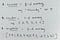# 5 Minutes of Machine Learning: Introduction to TensorFlow [Day 5]

Yes, and here we have it… the beginning of what Ashtanga yogis call the gatekeeper poses… (the hard ones that inevitably lead to lots of falling, but also lots of rewarding challenges when overcome)…

If you caught me here at the door of the gatekeeper things and are already scared shitless, please go back to my previous post in the 5 Minutes of Machine Learning series, or go to the first one and start there! It makes things slightly less scary.

So… TensorFlow!

Let’s jump right in.

# What is Tensorflow?

TensorFlow is a graph-based computational framework that can encode… anything you want it to.

TensorFlow (as most developers will interact with it) consists of low-level APIs that build models using mathematical operations. There are also high level APIs (such as functions like tf.estimator) for predefined architectures that can be used within TensorFlow (see my journey with this object classification and recognition model as an example of dealing with the high level APIs pre-crafted to complete certain known tasks).

There are two programming components to TensorFlow:

1. Graph protocol buffer.
2. The runtime to execute the graph.

What else is important to know about getting started programming with TensorFlow? Well, there is a hard way and an easy way (well okay, its not that binary, but you get it).

In TensorFlow, there are custom estimators, and there are pre-made estimators. What is the difference?

# Custom vs. Pre-Made Estimators in TensorFlow

Building and using a custom estimator in TensorFlow implies you write the model function yourself. In a pre-made model, someone already wrote the model function for you.

The sketch below (done by yours truly) seemed to clarify the difference between the two.

One of the thoughts that hit me pretty quickly about the difference between the two is the fact that even with custom estimators, you are still using pre-made estimators as building blocks in order to do two things:

1. Calculate a unique metric that has not already been built somewhere by someone.
2. Mess with hidden layers of “tensors”, connecting or adding them in unique ways.

I just used a strange word that is not so strange (because TensorFlow has it in the name… ) but let’s get a clearer idea of what a tensor really is now that we are talking specifically about TensorFlow.

# What is a Tensor?

A tensor is a primary data structure.

It can be N-dimensional, with N being a very, very large number. Tensors can be scalars, vectors, and/ or matrices (click on them to read more if you are unfamiliar with the algebra-ish terms). All three of these can hold integers, floating points, or string values.

So let’s look at these terms in context of TensorFlow with examples:Also keep in mind that matrices can go above a 2-D array.

Last but not least, what can be done to tensors? In my next post, I discuss how to create and manipulate tensors, but for now lets focus on basic CRUD operations (create, read, update, delete).

A graph’s nodes are equivalent to operations.

A graph’s edge = tensors.

Tensors flow through the graph, and are manipulated at each node by an operation.

This visualization is of an actual TensorFlow graph as it would appear on TensorBoard, TensorFlow’s analytics/ insights dashboard if you will that helps developers view the performance of their model training and inference processes: